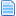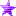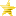Diagonal Lifebar on Character (Read 14212 times)

Started by -Extasis-, December 01, 2013, 04:07:49 pm

-Extasis-Diagonal Lifebar on Character
New #1  December 01, 2013, 04:07:49 pm
••• Tu tiempo paso, tu ya no brilla'♪♫
first explain the theory

Examplewe have to divide the straight parts of the diagonal3 explod will be to complete the bar

You should choose the percentage,each explod, I gave it a value equal to 3,       33% to 34% last

just change the formula of value
first explod left
Code:
`[State 1500,            VarSet]type                    = VarSettrigger1                = 1fv                      = 22value                   = ifelse((Fvar(21)-"Total life - percentage")<0,0,(Fvar(21)-"Total life - percentage"))/var(20)Second explod[State 1500,            VarSet]type                    = VarSettrigger1                = fvar(21)>= (life) - (the percentage given)fv                      = 23value                   = 0.25;full size of explod complete[State 1500,            VarSet]type                    = VarSettrigger1                = fvar(21)< (life) - (the percentage given)fv                      = 23value                   = ifelse((Fvar(21)-"percentage")<0,0,(Fvar(21)-"percentage"))/var(20)third right explod[State 1500,            VarSet]type                    = VarSettrigger1                = fvar(21)>=double maximum percentage subtracted  lifefv                      = 24value                   = 0.25[State 1500,            VarSet]type                    = VarSettrigger1                = fvar(21)<double maximum percentage subtracted  lifefv                      = 24value                   = ifelse((Fvar(21))<0,0,(Fvar(21)))/var(20)`

for the second explod
you need to create an explod trigger1 = 1, forever this being created, and removetime = -2, the animation should be 1 time

must divide the percentage of life, second explod in my case 33% = 333
in number, you decide my number is 4

333/4

create a variable for this only you have a animation of fightfx
F can not be used in anim ifelse the explod

Code:
`[State 1500,            VarSet]type                    = VarSettrigger1                = 1v                       = 19value                   = Ifelse(Fvar(21)>=584,0,Ifelse(Fvar(21)<=584&&Fvar(21)>=501,1,Ifelse(Fvar(21)<=501&&Fvar(21)>=418,2,Ifelse(Fvar(21)<=418&&Fvar(21)>=0,3,0))))[State 1500,            Explod]type                    = Explodtrigger1                = 1anim                    = F50024+var(19)ID                      = 50024pos                     = 101.4,20bindtime                = -1removetime              = -2facing                  = 1space                   = screenscale                   = 0.250,0.250sprpriority             = -11ontop                   = 0ownpal                  = 1ignorehitpause          = 1`

angles that give

Use this option only now photoshop or do-it Your Wayangles act this wayindeed between the diagonal is longer, more frames need

good luck

video

yo confio en que vuelvas a ser mi princesa ♥ ♪♫
Last Edit: December 01, 2013, 04:21:33 pm by -Extasis-